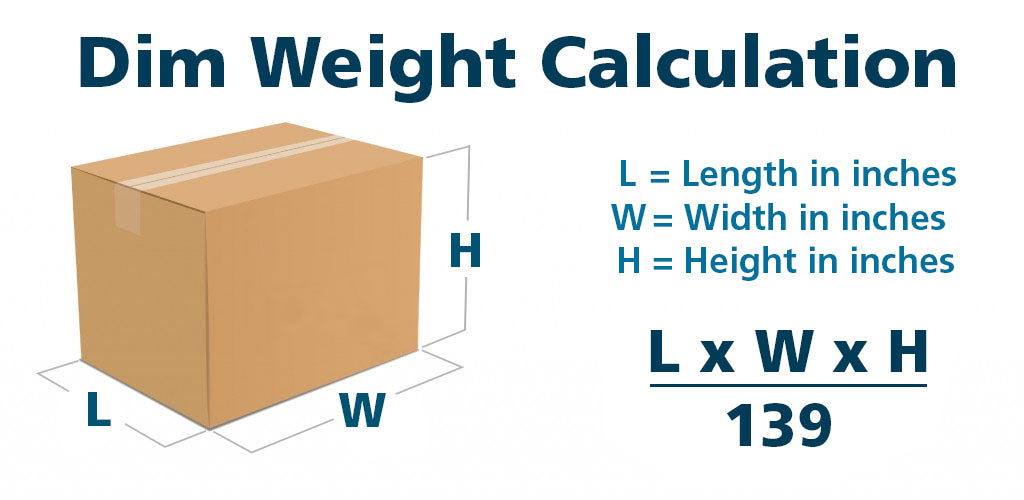What's the difference between actual weight and dimensional (DIM) weight? Like, why are some packages of light, corrugated plastic blanks calculated at a higher weight?

Shipping carriers consider actual weight to be the package weight, sometimes rounded up to the next whole pound if it falls in between. That's simple enough. Dimensional (DIM) weight, however, takes into consideration the amount of space a package occupies in relation to the actual weight of the package. Carriers compare the dimensional weight of a package to its actual weight and in most circumstances use the larger of the two to determine the billable weight. UPS, FedEx and US Postal Service all do this. DIM weight is the new normal. And starting January 2015, carriers will use the dimensional weight to calculate the cost of shipping ALL packages. Typical large items that cause issues are listed under 'Problem Children' below.

Here's how carriers determine dimensional (DIM) weight:

Determine the package dimensions in inches. For each dimension, measure at the longest point, rounding each measurement to the nearest whole number. For example, 1.00 to 1.49 would be considered 1, and 1.50 to 1.99 would be considered 2).Measure the length, width and height of the package at its extreme points. (If there's a bulge or the package is shaped irregularly, always include the bulge or irregular part of the package in the calculation.) Multiply the package length by the width by the height. (L x W x H) The result is the cubic size in inches.

For domestic ground shipments: If the cubic size of the package in inches is 1,728 or larger, divide the cubic size by 139 to determine dimensional weight in pounds. If the cubic size in inches is less than 1,728, use the divisor of 166. Increase any fraction to the next whole pound. Note: Starting January 2015, carriers will use dimensional weight instead of actual weight to calculate the cost of shipping ALL packages. UPS DIM Calc FedEx DIM Calc & Info

Why is understanding how carriers use DIM weight important? Sometimes the dimensions of the package override the actual weight and your shipment costs you and the company you're buying from a whole lot more. For example, here's how the amount of space the package occupies – not its actual weight – impacts the cost of shipping:

Ship Method / Destination: Ground / Domestic
Actual Weight: 32 pounds
Length: 30 inches
Width: 15 inches
Height: 15 inches
Cubic Size Calculation: 30 x 15 x 15 = 6,750 cubic inches
Dimensional Weight Calculation: 6,750/139 = 48.56 pounds, rounded up to 49 lbs

This package is being shipped domestic ground. It's cubic size in inches is divided by 139 to determine the dimensional weight, which is 49 pounds. The cubic size is larger than 1,728 cubic inches, so the dimensional weight of 49 pounds will be used as the billable weight – not the actual weight of 32 pounds.

Some typical 'Problem Children' that occupy a larger dimensional space, thereby overriding their actual weight and resulting in higher shipping costs:

24" x 18" and 36" x 24" Corrugated Plastic Sheets, Wind Sign II, Signicade, Squarecade, Econo Sign, Deluxe Signicade, Floor Racks and Wall Racks.